## September 21, 2014

### A Packing Pessimization Problem

#### Posted by John BaezIn a pessimization problem, you try to minimize the maximum value of some function:

$min_x max_y f(x,y)$

For example: which convex body in Euclidean space is the worst for packing? That is, which has the smallest maximum packing density?

(In case you’re wondering, we restrict attention to convex bodies because without this restriction the maximum packing density can be made as close to $0$ as we want.)

Of course the answer depends on the dimension. According to Martin Gardner, Stanislaw Ulam guessed that in 3 dimensions, the worst is the round ball. This is called Ulam’s packing conjecture.

In 3 dimensions, congruent balls can be packed with a density of

$\frac{\pi}{\sqrt{18}} = 0.74048048 \dots$

and Kepler’s conjecture, now a theorem, says that’s their maximum packing density. So, Ulam’s packing conjecture says we can pack congruent copies of any other convex body in $\mathbb{R}^3$ with a density above $\pi/\sqrt{18}$.

Ulam’s packing conjecture is still open. We know that the ball is a local pessimum for ‘centrally symmetric’ convex bodies in 3 dimensions. But that’s just a small first step.

Geometry is often easier in 2 dimensions… but in some ways, the packing pessimization problem for convex bodies in 2 dimensions is even more mysterious than in 3.

You see, in 2 dimensions the disk is not the worst. The disk has maximum packing density of

$\frac{\pi}{\sqrt{12}} = 0.9068996 \dots$

However, the densest packing of regular octagons has density

$\frac{4 + 4 \sqrt{2}}{5 + 4 \sqrt{2}} = 0.9061636 \dots$

This is a tiny bit less! So, the obvious 2-dimensional analogue of Ulam’s packing conjecture is false.

By the way, the densest packing of regular octagons is not the one with square symmetry:It’s this:which is closer in spirit to the densest packing of discs:The regular octagon is not the worst convex shape for packing the plane! Regular heptagons might be even worse. As far as I know, the densest known packing of regular heptagons is this ‘double lattice’ packing:studied by Greg Kuperberg and his father. They showed this was the densest packing of regular heptagons where they are arranged in two lattices, each consisting of translates of a given heptagon. And this packing has density

$\frac{2}{97}\left(-111 + 492 \cos\left(\frac{\pi}{7}\right) - 356 \cos^2 \left(\frac{\pi}{7}\right)\right) = 0.89269 \dots$

But I don’t know if this is the densest possible packing of regular heptagons.

Unlike the heptagon, the regular octagon is centrally symmetric: if we put its center at the origin, a point $x$ is in this region iff $-x$ is in the region.

The great thing about convex centrally symmetric regions of the plane is that their densest packing is always a lattice packing: you take your region $R$ and form a packing by using all its translates $R + L$ where $L \subseteq \mathbb{R}^2$ is a lattice. This is an old result of László Fejes Tóth and C. A. Rogers. For convex centrally symmetric regions, it reduces the search for the densest packing to a finite-dimensional maximization problem!

I said the regular octagon wasn’t the worst convex shape for densely tiling the plane. Then I said the regular heptagon might be worse, but I didn’t know. So what’s worse?

A certain smoothed octagon is worse:Since it’s centrally symmetric, we know its densest packing is a lattice packing, so it’s not miraculous that someone was able to work out its density:

$\frac{ 8-4\sqrt{2}-\ln{2} }{2\sqrt{2}-1} = 0.902414 \dots$

and the way it looks:In fact this shape is believed to be the worst centrally symmetric convex region for densely packing the plane! I don’t really know why. But Thomas Hales, who proved the Kepler conjecture, has an NSF grant based on a proposal where he says he’ll prove this:

In 1934, Reinhardt considered the problem of determining the shape of the centrally symmetric convex disk in the plane whose densest packing has the lowest density. In informal terms, if a contract requires a miser to make payment with a tray of identical gold coins filling the tray as densely as possible, and if the contract stipulates the coins to be convex and centrally symmetric, then what shape of coin should the miser choose in order to part with as little gold as possible? Reinhardt conjectured that the shape of the coin should be a smoothed octagon. The smoothed octagon is constructed by taking a regular octagon and clipping the corners with hyperbolic arcs. The density of the smoothed octagon is approximately 90 per cent. Work by previous researchers on this conjecture has tended to focus on special cases. Research of the PI gives a general analysis of the problem. It introduces a variational problem on the special linear group in two variables that captures the structure of the Reinhardt conjecture. An interesting feature of this problem is that the conjectured solution is not analytic, but only satisfies a Lipschitz condition. A second noteworthy feature of this problem is the presence of a nonlinear optimization problem in a finite number of variables, relating smoothed polygons to the conjecturally optimal smoothed octagon. The PI has previously completed many calculations related to the proof of the Reinhardt conjecture and proposes to complete the proof of the Reinhardt conjecture.

This research will solve a conjecture made in 1934 by Reinhardt about the convex shape in the plane whose optimal packing density is as small as possible. The significance of this proposal is found in its broader context. Here, three important fields of mathematical inquiry are brought to bear on a single problem: discrete geometry, nonsmooth variational analysis, and global nonlinear optimization. Problems concerning packings and density lie at the heart of discrete geometry and are closely connected with problems of the same nature that routinely arise in materials science. Variational problems and more generally control theory are have become indispensable tools in many disciplines, ranging from mathematical finance to robotic control. However, research that gives an exact nonsmooth solution is relatively rare, and this feature gives this project special interest among variational problem. This research is also expected to further develop methods that use computers to obtain exact global solutions to nonlinear optimization problems. Applications of nonlinear optimization are abundant throughout science and arise naturally whenever a best choice is sought among a system with finitely many parameters. Methods that use computers to find exact solutions thus have the potential of finding widespread use. Thus, by studying this particular packing problem, mathematical tools may be further developed with promising prospects of broad application throughout the sciences.

I don’t have the know-how to guess what Hales will do. I haven’t even read the proof of that theorem by László Fejes Tóth and C. A. Rogers! It seems like a miracle to me.

But here are some interesting things that it implies.

Let’s say a region is a relatively compact open set. Just for now, let’s say a shape is a nonempty convex centrally symmetric region in the plane, centered at the origin. Let $Shapes$ be the set of shapes. Let $Lattices$ be the set of lattices in the plane, where a lattice is a discrete subgroup isomorphic to $\mathbb{Z}^2$.

We can define a function

$density: Shapes \times Lattices \to [0,1]$

as follows. For each shape $S \in Shapes$ and lattice $L \in Lattices$, if we rescale $S$ by a sufficiently small constant, the resulting shape $\alpha S$ will have the property that the regions $\alpha S + \ell$ are disjoint as $\ell$ ranges over $L \subseteq \mathbb{R}^2$. So, for small enough $\alpha$, $\alpha S + L$ will be a way of packing the plane by rescaled copies of $S$. We can take the supremum of the density of $\alpha S + L$ over such $\alpha$, and call it

$density(S,L)$

Thanks to the theorem of László Fejes Tóth and C. A. Rogers, the maximum packing density of the shape $S$ is

$\sup_{L \in Lattices} density(S,L)$

Here I’m taking advantage of the obvious fact that the maximum packing density of $S$ equals that of any rescaled version $\alpha S$. And in using the word ‘maximum’, I’m also taking for granted that the supremum is actually attained.

Given all this, the pessimization problem for packing centrally symmetric convex regions is all about finding

$\inf_{S \in Shapes} \; \sup_{L \in Lattices} density(S,L)$

But there’s more nice symmetry at work here. Linear transformations of the plane act on shapes, and lattices, and packings… and the concept of density is invariant under linear transformations!

One thing this instantly implies is that the maximum packing density for a centrally symmetric convex region doesn’t change if we apply a linear transformation to that region.

This is quite surprising. You might think that stretching or shearing a region could give a radically new way to pack it as densely as possible. And indeed that’s probably true in general. But for centrally symmetric convex regions, the densest packings are all lattice packings. So if we stretch or shear the region, we can just stretch or shear the lattice packing that works best, and get the lattice packing that works best for the stretched or sheared region. The packing density is unchanged!

We can say this using jargon. The group of linear transformations of the plane is $GL(2, \mathbb{R})$. This acts on $Shapes$ and $Lattices$, and for any $g \in GL(2, \mathbb{R})$ we have

$density(g S, g L) = density(S,L)$

So, the function

$density: Shapes \times Lattices \to [0,1]$

is $GL(2,\mathbb{R})$-invariant. And thus, the maximum packing density is invariant:

$\sup_{L \in Lattices} density(S,L) = \sup_{L \in Lattices} density(g S,L)$

for all $g \in GL(2,\mathbb{R})$.

As mentioned before, we also have

$density(\alpha S, L) = density(S, L)$

where $\alpha$ is any nonzero scalar multiple of the identity matrix (and thus a rescaling if $\alpha > 0$). So, we can replace $Shapes$ by the quotient spaces $Shapes/\mathbb{R}^*$, and work with

$density: Shapes/\mathbb{R}^* \times Lattices \to [0,1]$

$GL(2,\mathbb{R})$ still acts on the first factor here, with scalar multiples of the identity acting trivially, and this map is still $GL(2,\mathbb{R})$-invariant.

I think there should be a topology on $Shapes$ that makes the quotient space $Shapes/\mathbb{R}^*$ compact and makes

$density: Shapes \times Lattices \to [0,1]$

continuous. Something like the Hausdorff metric, maybe. Can anyone help me here?

None of this goes far in solving the packing pessimization problem for convex centrally symmetric regions in the plane. We’ve reduced the number of degrees of freedom, but they’re still infinite.

But still, it’s fun. I like how it’s vaguely reminiscent of the theory of modular functions, which can be seen as $SL(2,\mathbb{R})$-invariant functions of a lattice together with an ellipse centered at the origin.

For more on packing pessimization problems, see:

To see who drew the pretty pictures, click on the pictures.

There’s a lot of good stuff in the comments. Most notably:

• The set $Shapes/GL(2,\mathbb{R})$ has a nice topology making it a compact space. This is the moduli space of 2-dimensional real Banach spaces! In general, the moduli space of $n$-dimensional real Banach spaces is called a Banach–Mazur compactum; click the link for details on its topology.

• Amazingly, there is a one-parameter family of maximally dense packings of the smoothed octagon! Greg Egan has made a movie showing all these packings: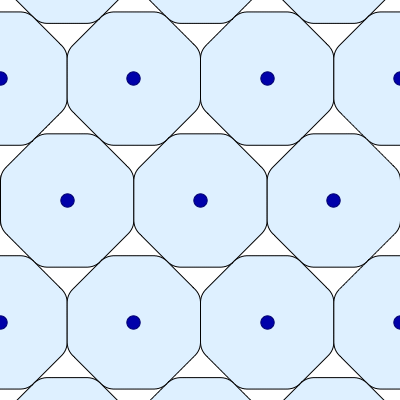As the smoothed octagons turn, their centers move but the area in each space between them stays the same!

Posted at September 21, 2014 4:38 PM UTC

TrackBack URL for this Entry:   https://golem.ph.utexas.edu/cgi-bin/MT-3.0/dxy-tb.fcgi/2768

### Re: A Packing Pessimization Problem

The article “The 3-ball is a local pessimum for packing” is published here behind a paywall. But the arXiv version of the article is clearly more useful :-)

Posted by: David Roberts on September 22, 2014 7:49 AM | Permalink | Reply to this

### Re: A Packing Pessimization Problem

I just realised something very nice about the smoothed octagon! I was wondering why you couldn’t reduce its packing density any further by slicing a small piece off each corner, but then I calculated the density if you line up the octagons vertex-to-vertex in rows, instead of edge-to-edge.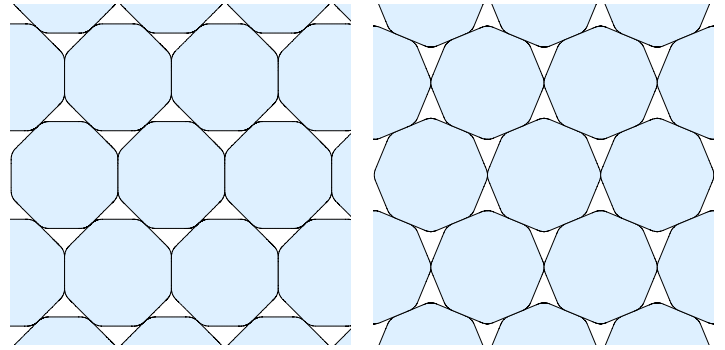These two packings turn out to have precisely the same density! So if you cut a bit off the corners, although you would reduce the density of the first packing, you would actually increase the density of the second packing (if you squeezed it horizontally so the figures made contact again after the cut).

Posted by: Greg Egan on September 22, 2014 1:11 PM | Permalink | Reply to this

### Re: A Packing Pessimization Problem

Cool! Now I’m guessing there’s actually a continuous 1-parameter way to turn all those smoothed octagons from the first position you show to the second, while keeping their density constant.

Anyway, that’s my interpretation of the pictures from page 13 to page 19 here:

Posted by: John Baez on September 22, 2014 9:41 PM | Permalink | Reply to this

### Re: A Packing Pessimization Problem

Okay, in this paper I mentioned:

he writes:

Reinhardt’s smoothed octagon possesses the property that its lattice packing density is achieved simultaneously by a one-parameter family of lattices (see Figure 1). In fact this property, common to all so-called irreducible domains (domains all of whose proper subdomains have admissible lattices of lower mean area), has long been a central organizing idea in the study of Reinhardt’s conjecture . Therefore, it might be surprising to some that the heptagon, despite being irreducible with respect to double lattices, does not have a one parameter family of optimal admissible double lattices.

Here is part of Figure 1: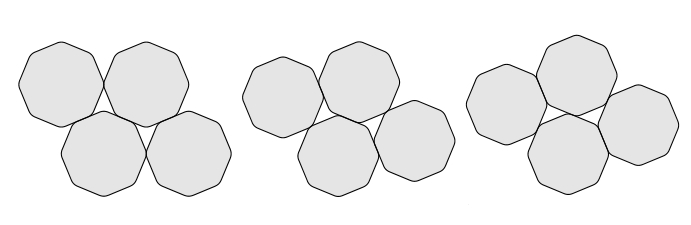This property of smoothed octagons is clearly ‘miraculous’, and this miracle goes a long way toward persuading me that they might pessimize the packing density, even though I don’t see any logical connection! Before, I hadn’t seen what was special about them.

Posted by: John Baez on September 22, 2014 10:00 PM | Permalink | Reply to this

### Re: A Packing Pessimization Problem

I wrote:

… even though I don’t see any logical connection!

I take that back! Greg wrote:

So if you cut a bit off the corners, although you would reduce the density of the first packing, you would actually increase the density of the second packing (if you squeezed it horizontally so the figures made contact again after the cut).

Presumably with even more equal-density packings, this sort of argument puts even more constraints on how we could tweak the smoothed octagon to get a shape with lower packing density!

It has been proved that the smoothed octagon is a local pessimum for packing density of centrally symmetric convex regions:

• F. L. Nazarov, On the Reinhardt problem of lattice packings of convex regions: local extremality of the Reinhardt octagon, J. Soviet Math. 43 (1988), 2687.

Maybe he used this sort of argument; I haven’t read the paper.

Posted by: John Baez on September 22, 2014 10:10 PM | Permalink | Reply to this

### Re: A Packing Pessimization Problem

Ah, I’d skimmed that part of Kallus’s paper a couple of days ago, and then I managed to forget exactly what he was saying there! The next thing I was going to try to prove was that you could find packings of the smoothed octagon with equal densities that included points of contact at every point on the perimeter. Though Kallus doesn’t quite say that explicitly, I’m sure that’s what’s going on here.

Posted by: Greg Egan on September 22, 2014 11:21 PM | Permalink | Reply to this

### Re: A Packing Pessimization Problem

Since you like making animated movies of mathematically interesting gears, I think your mission here is clear.But I guess the lattice points on which the smoothed octagons are centered must move as the smooth octagons rotate? That would make all these ‘gears’ — except perhaps for a central fixed one — shift around in a disconcerting way as they turn.

But that could be interesting…

For those who haven’t been following Greg’s recent work on higher-dimensional gears and group representation theory, click here:Posted by: John Baez on September 23, 2014 12:49 AM | Permalink | Reply to this

### Re: A Packing Pessimization ProblemThe motion of the lattice centres is quite disconcerting! But it does look plausible that those triangular-ish gaps are maintaining a constant area throughout the cycle.

Posted by: Greg Egan on September 23, 2014 3:58 AM | Permalink | Reply to this

### Re: A Packing Pessimization Problem

Wow, that’s great! As I feared, there’s something about the sloshing motion that makes me a bit sea-sick. But it’s quite an amazing setup: ‘gears’ that would work with an incompressible fluid between them!

Posted by: John Baez on September 23, 2014 4:47 AM | Permalink | Reply to this

### Re: A Packing Pessimization Problem

Never mind the gaps; it’s enough to work out what the blue-vertex triangles are doing.

Posted by: Jesse C. McKeown on September 23, 2014 5:09 AM | Permalink | Reply to this

### Re: A Packing Pessimization Problem

… because if the areas of those triangles are constant, and the areas of the rounded octagons are constant (which they are), the areas of the gaps are constant?

It’s a little hard for me to see that. I guess at every moment, each triangle contains one whole gap and half the area of an octagon.Posted by: John Baez on September 23, 2014 3:00 PM | Permalink | Reply to this

### Re: A Packing Pessimization Problem

John wrote:

It’s a little hard for me to see that. I guess at every moment, each triangle contains one whole gap and half the area of an octagon.

It’s hard for me to visualise the precise contents of the triangles, because everything is in motion and the edges of the triangles aren’t marked. But given the fixed area of the octagons, a constant area for the triangles guarantees a constant packing density, which in turns implies a constant area for the gaps.

Posted by: Greg Egan on September 23, 2014 3:03 PM | Permalink | Reply to this

### Re: A Packing Pessimization Problem

Good point, Jesse! That’s an easier calculation than the areas of the gaps.

Numerically, I do get a constant result for the areas of the triangles. That is, the area of the fundamental domain of the lattice remains the same as the octagon rotates.

I haven’t ploughed through all the algebra yet to prove that this is true, but it’s clear now that this is what Reinhardt must have proved (or something equivalent).

Posted by: Greg Egan on September 23, 2014 6:54 AM | Permalink | Reply to this

### Re: A Packing Pessimization Problem

I checked algebraically, and the areas of the lattice triangles are constant!

The calculations I have at present are too messy to reproduce here. There’s probably a nicer way of proving this than grinding through the algebra, based on some simple geometric properties of the smoothed octagon.

Posted by: Greg Egan on September 23, 2014 9:35 AM | Permalink | Reply to this

### Re: A Packing Pessimization Problem

@johnbaez: the space of (linear) isometry classes of $n$-dimensional normed spaces is a compact one, called the Banach-Mazur compactum. This is the same as the quotient of Shapes by $GL(2,\mathbb{R})$. But if you quotient only by scalars, the space is non-compact, as shapes of volume $1$, say, can degenerate to a line.

Posted by: bruno on September 22, 2014 3:41 PM | Permalink | Reply to this

### Re: A Packing Pessimization Problem

Great, thanks! I’ll have to learn about the Banach–Mazur compactum; it sounds like a cool gadget.

If it’s really the quotient $Shapes/GL(2,\mathbb{R})$, we should be able to define the maximum packing density as a continuous function on it and use its compactness to see various things — for example, that this function has a minimum.

Posted by: John Baez on September 22, 2014 9:34 PM | Permalink | Reply to this

### Re: A Packing Pessimization Problem

I also think it’s great that the space I was calling $Shapes$ is the moduli space of 2-dimensional Banach spaces. This makes it seem much more like a reputable mathematical object.

The point, of course, is that what I was calling a ‘shape’ is precisely the same as a unit ball for some norm on $\mathbb{R}^2$.

So, the packing pessimization problem I was discussing here, for which the smoothed octagon is the conjectured answer, can be rephrased as:

“find a norm on $\mathbb{R}^2$ that minimizes the maximum packing density”

where the packing density of a lattice $L \subseteq \mathbb{R}^2$ is the density of points that have distance $\le 1$ from some point in $L$, and the maximum packing density is maximum value of the packing density over all lattices $L \subseteq \mathbb{R}^2$ for which the nonzero lattice points have norm $\ge 2$.

And since we’re at the $n$-Category Café, I should mention that there’s also an interesting moduli stack of $n$-dimensional Banach spaces. The ‘stacky points’ are the Banach spaces with extra symmetry. Well, every Banach space has the symmetry $x \mapsto -x$, so there’s a kind of $\mathbb{Z}_2$ stackiness all over the place, but some Banach spaces have more symmetry. For example, the one corresponding to the smoothed octagon has a 16-element dihedral group acting as symmetries:Posted by: John Baez on September 23, 2014 1:14 AM | Permalink | Reply to this

### Re: A Packing Pessimization Problem

Just for fun, here’s a version of the rotating-smoothed-octagon lattice annotated with a “squaring of the triangle”. The area of the lattice triangle is transferred to the area of the yellow square, and the fact that this square remains unchanged reflects the fact that the lattice triangle has a constant area even as the smoothed octagons rotate.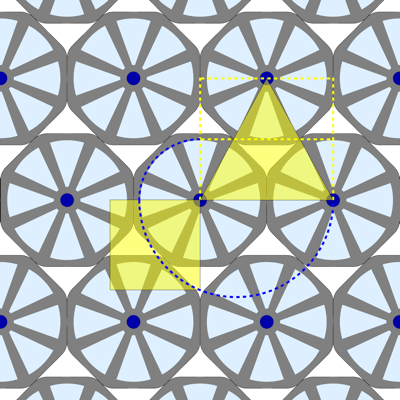Posted by: Greg Egan on September 23, 2014 12:00 PM | Permalink | Reply to this

### Re: A Packing Pessimization Problem

Thanks for this extra visualization, Greg, it definitely helps nail down the constant areas of the gaps. But I confess that my intuition is still failing me a little bit. I think it would help to see a bunch more rows of this rolling configuration. Do you think you’d be willing to produce a zoomed-out version of this animation? Or even an interactive version, though I recognize that’s probably more hackery than is really worthwhile…

Posted by: Craig Kaplan on September 24, 2014 3:49 AM | Permalink | Reply to this

### Re: A Packing Pessimization Problem

Craig, here’s a version that’s zoomed out by a factor of three.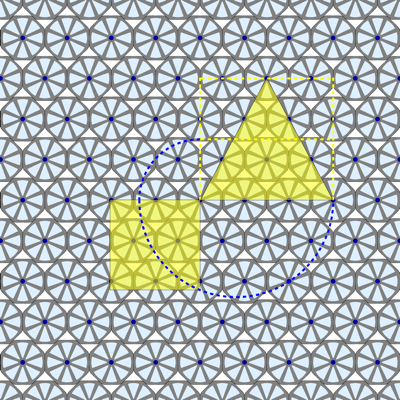Posted by: Greg Egan on September 24, 2014 4:58 AM | Permalink | Reply to this

### Re: A Packing Pessimization Problem

Greg, that’s a great visualization. Can you explain how you know the square has the same area as the triangle? Am I missing something obvious?

Posted by: Simon Willerton on September 24, 2014 9:09 AM | Permalink | Reply to this

### Re: A Packing Pessimization Problem

Call the base of the triangle $b$ and the height of the triangle $h$.

The quarter-circle has radius $r_1=\frac{h}{2}$, while the semi-circle has radius $r_2=\frac{b+r_1}{2}$. The top right corner of the square is constructed to be a distance of $r_2-r_1$ from the centre of the semicircle, while the bottom right corner, lying on the semi-circle, is $r_2$ from the centre. So by Pythagoras, the area of the square is:

$A = r_2^2 - (r_2-r_1)^2 = r_1 (2 r_2-r_1) = \frac{1}{2} h b$

Posted by: Greg Egan on September 24, 2014 10:36 AM | Permalink | Reply to this

### Re: A Packing Pessimization Problem

Cunning! I hadn’t realised that it was a quarter-circle and a half-circle: I’d assumed it was some kind of mystical spiral. Thanks.

Posted by: Simon Willerton on September 24, 2014 12:53 PM | Permalink | Reply to this

### Re: A Packing Pessimization Problem

Now I’ve realized there’s a nice analogy

sphere packing problems :
lattices in vector spaces with an inner product ::
shape packing problems :
lattices in vector spaces with a norm

My vector spaces are finite-dimensional and real. I’m using shape to mean an open ball in a normed vector space: this is equivalent to my earlier definition where I said a shape was a relatively compact open set that is convex and centrally symmetric. And in this analogy I’m only considering lattice packings, where the spheres or shapes are centered at points in a lattice.

Say we have a lattice $L$ in a vector space with an inner product. We can pack unit balls centered at points of $L$ if the shortest nonzero vector $\ell \in L$ has length $\ge 2$. If its length is exactly $2$, the packing is ‘tight’: we can’t expand all the spheres without them overlapping.

All this works equally well if our vector space is equipped with a more general norm, not necessarily coming from an inner product. Now the balls don’t look round: they are more general shapes!

So, the ‘analogy’ above is really a generalization.

Posted by: John Baez on September 23, 2014 3:26 PM | Permalink | Reply to this

### Re: A Packing Pessimization Problem

The point of my last comment is that there’s a big theory relating modular forms to vector spaces equipped with a lattice and an inner product, with applications to sphere packings. A good references is Sphere Packings, Lattices and Groups by Conway and Sloane. It would be interesting to see if we could generalize any of this to vector spaces equipped with a lattice and a norm, and apply it to shape packings.

This would be quite a challenge, since a bunch of things don’t easily generalize. For example, consider the theta function of a lattice equipped with an inner product:

$\sum_{\ell \in L} e^{i \pi \tau \langle \ell, \ell \rangle}$

where $\tau \in \mathbb{C}$ lies in the upper half-plane. This has lots of great properties that won’t generalize if we replace $\langle \ell, \ell \rangle$ by $\|\ell\|^2$ where the norm doesn’t come from an inner product.

Nonetheless I think this project is worth looking into.

Posted by: John Baez on September 23, 2014 4:44 PM | Permalink | Reply to this

### Re: A Packing Pessimization Problem

In case it’s not obvious, we can take any (finite-dimensional real) normed vector space containing a lattice $L$, form the theta function

$\Theta_L(\tau) = \sum_{\ell \in L} e^{i \pi \tau \|\ell\|^2}$

and show it converges for $\tau$ in the upper half-plane. Then the usual trick of writing

$q = e^{2 \pi i \tau}$

gives

$\Theta_L(\tau) = \sum_{\ell \in L} q^{\frac{1}{2}\|\ell\|^2} ,$

so the coefficient of $q^{s/2}$ in this power series counts the number of vectors in $L$ having norm $\sqrt{s}$.

This is standard stuff when our norm comes from an inner product, and it still works.

However, the fun usually starts when the norm comes from an inner product and the inner product of any two vectors in $L$ is an integer! Then $\Theta_L(\tau)$ is a modular form, and we can do tons of fun stuff. Most of that stuff appears to be denied to us now. But maybe there is new, harder stuff.

In the ordinary case when our norm comes from an inner product (aka quadratic form), does anyone know a formula to recover the density of some associated sphere packing from theta function $\Theta_L(\tau)$?

Posted by: John Baez on September 23, 2014 10:06 PM | Permalink | Reply to this

### Re: A Packing Pessimization Problem

I wrote:

In the ordinary case when our norm comes from an inner product (aka quadratic form), does anyone know a formula to recover the density of some associated sphere packing from theta function $\Theta_L(\tau)$?

I bet the answer to this underlies the Minkowski–Hlawka bound that I mentioned. Here’s a way to get started.

We have a vector space with a lattice $L$ and a norm $\|\cdot \|$. Rescale one of them so that the shortest nonzero vector in the lattice has length $2$. Then $L$ gives a way of packing unit balls:

$\{x : \|x - \ell\| \le 1 \} \qquad \ell \in L$

Warning: these balls may not be round, since the norm $\| \cdot \|$ may be funny: they’re just ‘shapes’ in the technical sense I’ve defined earlier. In the 2d case they could be squares, or octagons, or smoothed octagons, etc.

Let’s calculate the density of this packing. We’ll do this by considering a huge ball of radius $R$ and counting the number $n(R)$ of lattice points inside it. Up to some small error term, the density will be $n(R)$ times the volume of a unit ball divided by the volume of the big ball. The error term will go to zero as $R \to \infty$.

Let $V$ be the volume of a unit ball. Then by what I said, the density will be

$density = \lim_{R \to \infty} \frac{n(R) V}{R^d V}$

where $d$ is the dimension of our vector space. The volume of the unit ball cancels out, so we get

$density = \lim_{R \to \infty} \frac{n(R)}{R^d}$

So, how do we calculate $n(R)$? Since I’m trying to generalize the usual relation between lattices and modular forms, I want to use the ‘theta function’ of the lattice. I’ll switch to a better convention where this is defined to be

$\theta_L(q) = \sum_{\ell \in L} q^{\|\ell\|^2}$

getting rid of a $\frac{1}{2}$ that infests the usual definition.

The point is now that the coefficient of $q^s$ is the number of vectors in $L$ with norm $\sqrt{s}$. So, to calculate $n(R)$, we ‘just’ take the theta function and sum the coefficients of $q^s$ for all $s$ with $s \le r^2$.

This just shows that the density of the packing can in principle be recovered from the theta function of the lattice. It’s not very practical until we can more easily do what I said we should ‘just’ do.

I’m guessing Minkowski was smart enough to extract practical consequences from this idea.

Posted by: John Baez on September 25, 2014 12:44 AM | Permalink | Reply to this

### Re: A Packing Pessimization Problem

In my somewhat crazy quest to related packings of general nonspherical shapes to modular forms, I’ve run across this one tantalizing clue:

Overview of sphere-packing bounds; theta series of unimodular lattices; extremal theta functions and lattices

The analogue for sphere packings of the Gilbert-Varshamov bound [for codes] is the Minkowski–Hlawka bound, which holds for packings not just of spheres but of translates of an arbitrary centrally-symmetric convex body $B$ in $\mathbb{R}^n$, as long as $B$ is bounded and has positive volume.

from Noam Elkies’ webpage on Math 256x: The Theory of Error-Correcting Codes (Fall 2013).

A centrally-symmetric convex body obeying these conditions amounts to precisely what I’ve been calling a ‘shape’: a ball in some finite-dimensional real normed vector space. And the Minkowski–Hlawka bound says that for any shape in dimension $n$ there is a lattice packing with density at least

$\frac{\zeta(n)}{2^{n-1}}$

The interesting thing about this bound is that it does not give a way to construct a packing this dense. And as Elkies mentions,

it is a long-standing embarrassment that we cannot attain this bound “explicitly”, nor do substantially better, even for Euclidean spheres.

So, for spheres in high-dimensional Euclidean spaces, we know it’s possible to pack them much more densely than anyone has!

I hadn’t know this stuff applies to more general shapes, and now I’m wanting to see how the proof goes and whether it has anything to do with theta functions.

Posted by: John Baez on September 23, 2014 10:25 PM | Permalink | Reply to this

### Re: A Packing Pessimization Problem

Just for the sake of completeness, here’s an earlier version of Greg Egan’s movie of rolling smoothed octagons:You have to work harder to see that the octagons are rolling, but it has a charm all its own.

Posted by: John Baez on September 23, 2014 10:53 PM | Permalink | Reply to this

### Re: A Packing Pessimization Problem

Here’s a nice way to construct the corners of the smoothed octagons.

Start out with unsmoothed regular octagons. Arrange three of them in their closest packing: two of them lined up horizontally, sharing an edge, and the third one midway between them horizontally, and elevated vertically just enough to sit neatly in the gap between the first two.

Then start rotating all three octagons. Keep the $y$ coordinate of the two in the bottom row fixed, and adjust the distance between them so their edges stay in contact. Position the top octagon so it has one edge flush against an edge of the bottom-left octagon, and choose the $y$ coordinate of the top octagon’s centre so that the triangle formed by all three centres has a constant area.

The problem this configuration creates for ordinary octagons is that the top octagon and the bottom-right octagon will start to overlap, close to a certain pair of vertices. But the solution is simple! Take the midpoint between those two vertices, and use it to trace out a curve. This curve becomes the new boundary of the octagons, smoothing away the original corners and guaranteeing that there is no overlap. Then just repeat this curve at all the other vertices, by symmetry.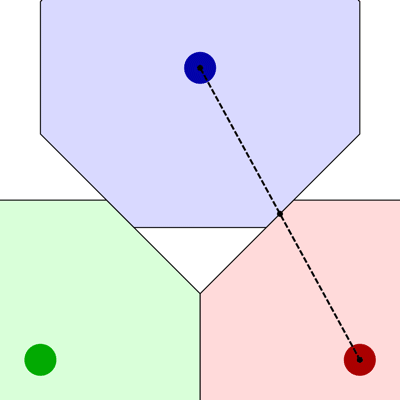This is what it looks like in close-up: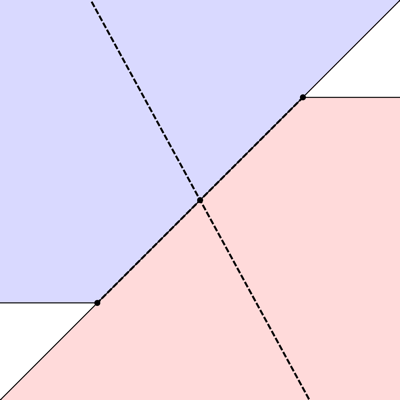Posted by: Greg Egan on September 25, 2014 2:39 AM | Permalink | Reply to this

### Re: A Packing Pessimization Problem

Actually, it’s even simpler than I put it.

The point that traces out the smoothed corner is midway between the two vertices that are involved in the overlap of the octagons. But the whole setup is so symmetrical that this point is also midway between the centres of the two octagons in question. That makes it much clearer that there’s no overlap as the smoothed figures rotate: the borders here are simply defined to split the difference in the distance between the octagons’ centres.

Posted by: Greg Egan on September 25, 2014 4:43 AM | Permalink | Reply to this

### Re: A Packing Pessimization Problem

Here’s a derivation of the shape of the smoothed octagon’s corners, starting from the assumption that the packing density remains constant as the figure rotates.

Suppose we begin with regular octagons of width 2. That is, the distance from the centre to the midpoint of each edge is 1. Then if we rotate the octagons shown in the animation in the previous comment by an angle $\theta$, keeping the bottom-left octagon’s centre fixed at $(0,0)$, the bottom-right octagon’s centre will move to $(2 \sec(\theta),0)$ in order for the edges to continue to make contact along a line segment.

The top octagon’s centre will move to:

$\left(\frac{\sec(\theta+\pi/4)}{\sqrt{2}} - \left(2\sqrt{2}-1\right)\sin(\theta), \left(2\sqrt{2}-1\right)\cos(\theta)\right)$

in order that it also has an edge that lies flush against one of the bottom-left octagon’s edges, and that the area of the triangle formed by the three octagons’ centres remains constant.

A vector that goes halfway from the top octagon’s centre to the bottom-right octagon’s centre is given by:

$P=\left(\sec(\theta)-\frac{\sec(\theta+\pi/4)}{2\sqrt{2}}+\left(\sqrt{2}-\frac{1}{2}\right)\sin(\theta),-\left(\sqrt{2}-\frac{1}{2}\right)\cos(\theta)\right)$

This takes us to the point that traces out the smoothed corner of the octagon.

If we re-express $P$ in a basis that rotates along with the top octagon, with our new x-axis pointing to the vertex that overlaps with the bottom-right octagon, and we change our parameter from $\theta$ to $t=\tan(\theta)$, we obtain:

$x(t) = \cos \left(\frac{\pi }{8}\right) t+\frac{\csc \left(\frac{\pi }{8}\right)}{4 (t-1)}+\sin \left(\frac{\pi }{8}\right)+\frac{1}{2} \csc \left(\frac{\pi }{8}\right)$ $y(t) = -\sin \left(\frac{\pi }{8}\right) t +\frac{\left(\sqrt{2}-1\right) \csc \left(\frac{\pi }{8}\right)}{4 (t-1)}+\sin \left(\frac{\pi }{8}\right)$

It’s not hard to eliminate $t$ from these equations, which gives us the hyperbola:

$y^2 = \left(3-2 \sqrt{2}\right) \left(x-\csc \left(\frac{\pi }{8}\right)\right)^2-\sqrt{2}+1$

As we follow the parameterised curve from $t=0$ to $t=1-\frac{1}{\sqrt{2}}$, $x(t)$ returns to its starting value, and $y(t)$ to the opposite of its starting value, completing the corner.

We can’t continue to use the same functions of $\theta$ as the coordinates for the centres of the three octagons for larger values of $\theta$ than $\tan ^{-1}\left(1-\frac{1}{\sqrt{2}}\right)$, because beyond that point the bottom octagons begin to make contact along smoothed corners rather than the original flat edges. But the shape we’ve found for those smoothed corners and the symmetry of the figures guarantees that we can maintain the constant area of the triangle formed by the three octagon centres, as $\theta$ continues through a full rotation.

Posted by: Greg Egan on September 25, 2014 8:40 AM | Permalink | Reply to this

### Re: A Packing Pessimization Problem

This is great, Greg! This construction gives a much clearer way to define the smoothed octagon, if the goal is to understand its interesting properties. At some point you or I could edit the Wikipedia article smoothed octagon and improve it. An animated gif or two would help it.

Posted by: John Baez on September 25, 2014 7:46 PM | Permalink | Reply to this

### Re: A Packing Pessimization Problem

OK, I’ve added a description of the corner construction to the Wikipedia article (with an animated illustration, but no algebraic details – I left in all the details of the hyperbola that were already in the article).

Posted by: Greg Egan on September 26, 2014 5:39 AM | Permalink | Reply to this

### Re: A Packing Pessimization Problem

Thanks a lot! I like how your figure matches the existing one in color and has the central octagon in a fixed orientation. I made a microscopic change in the wording of the article.

Posted by: John Baez on September 27, 2014 6:58 AM | Permalink | Reply to this

### Re: A Packing Pessimization Problem

It took me a bit of pondering to understand how to do that diagram efficiently!

In my previous animations where I rotated all the octagons, once you’ve rotated by $\pi/4$ everything looks identical and you can close the loop. But if you use a basis where the orientations of the octagons are fixed but they move around the central one, rotating by $\pi/4$ gives you an isomorphic packing, but not an identical one. It wasn’t until I did all the calculations described here that I could exploit the six-fold “symmetry” and create a loop in which each of the six nearest neighbours of the central octagon moves into the original position of its own neighbour in that set of six.

Posted by: Greg Egan on September 27, 2014 12:11 PM | Permalink | Reply to this

### Re: A Packing Pessimization Problem

There are many examples of centrally symmetric convex bodies whose optimal packings form a 1-dimensional family. The smoothed octagon is not at all unique in this regard.

For example, you can continuously deform the circle into a smoothed octagon in such a way that every intermediate convex body optimally packs in a 1-dimensional family.

Every curve in $\mathrm{SL}_2(\mathbb{R})$ (subject to some mild constraints) can be used to construct such a centrally symmetric body. The circle corresponds to a curve with image $\mathrm{SO}(2)$ in $\mathrm{SL}_2(\mathbb{R})$. A different curve in $\mathrm{SL}_2(\mathbb{R})$ produces the smoothed octagon.

To go from a curve $\phi:[t_0,t_1] \to \mathrm{SL}_2(\mathbb{R})$ to a convex body, let $u_1,..,u_6$ be the vertices of a regular hexagon centered at 0 in the plane. Then the six curves $\phi(t) u_1,...$ simultaneously trace out different arcs along the boundary of the body. You need to fit boundary conditions so that $\phi(t_1)u_1$ matches smoothly with $\phi(t_0)u_2$. The boundary conditions on the other arcs are then automatic. Not all choices of $\phi$ give a convex boundary, but this is easy to arrange. The “corner smoothing” happens automatically in this construction.

I’m sure these other examples will make you seasick in the same wayas Egan’s animations.

Posted by: Tom Hales on September 25, 2014 7:42 AM | Permalink | Reply to this

### Re: A Packing Pessimization Problem

That’s intriguing! Is it correct that this path $\phi:[t_0,t_1]\to SL(2, \mathbb{R})$ will map a basis for the $A_2$ lattice into the 1-parameter family of optimal packings for the convex body that $\phi$ generates from the hexagon vertices?

Posted by: Greg Egan on September 25, 2014 10:52 AM | Permalink | Reply to this

### Re: A Packing Pessimization Problem

Thomas wrote:

For example, you can continuously deform the circle into a smoothed octagon in such a way that every intermediate convex body optimally packs in a 1-dimensional family.

Nice! Watching this slowly happen while having each body rotate would be fun to see: at first it would look like nothing is happening, since a rotated circle is a circle, but at the end we’d get this:But this would probably be unreasonably long for an animated gif.

Thanks for stopping by, and good luck on your pessimal packing project! You really tackle some hard geometry problems.

Posted by: John Baez on September 27, 2014 8:46 AM | Permalink | Reply to this

### Re: A Packing Pessimization Problem

That is right. If you draw the six tangents to the six curves at any time t, you get a centrally symmetric hexagon that tiles the plane, giving the translates of the convex body in an optimal packing.

Posted by: Tom Hales on September 25, 2014 12:57 PM | Permalink | Reply to this

### Re: A Packing Pessimization Problem

I don’t understand how a hexagon built from the tangents to the six curves can yield an optimal packing of the convex body.

Suppose $\phi:[t_0,t_1]\to\mathrm{SL}_2(\mathbb{R})$, and we construct the convex body from the six arcs $\phi(t) v_i, t\in[t_0,t_1]$, where the six vectors $v_i$ are the shortest non-zero vectors in the $A_2$ lattice, which lie on the vertices of a regular hexagon.

Then, at least for the example of the smoothed octagon, the 1-parameter family of optimal packings can be found using $2 \phi(t) A_2$ as the lattice. That’s what I used here: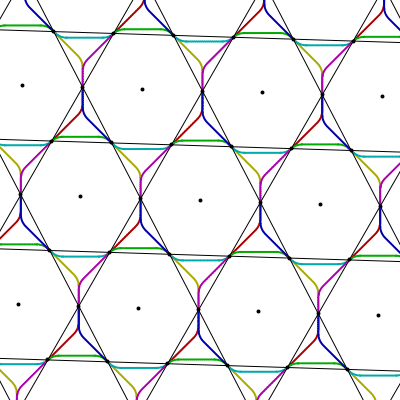In this construction, for a given value of the parameter $t$, the copy of the smoothed octagon centred at the origin makes contact with six neighbours whose centres are found simply by doubling the six vectors $\phi(t) v_i$, which lie on the perimeter of the octagon at the origin.

But I can’t see how the six tangents $\phi'(t) v_i$ relate to this. I’ve tried constructing a hexagon from these tangents and packing the plane with that hexagon, but when I do this, neither the centres nor the vertices of that packing (multiplied by any scaling factor) seem to form a lattice that gives an optimal packing for the smoothed octagon.

Posted by: Greg Egan on September 29, 2014 3:28 AM | Permalink | Reply to this

### Re: A Packing Pessimization Problem

Oh, I was being obtuse, I understand now!

I was constructing hexagons in the tangent space, i.e. hexagons whose vertices were (proportional to) the tangent vectors to the six curves. I thought there was some mysterious duality going on that would make sense of this, but there wasn’t, I was just missing the point entirely.

The tangents that Thomas Hales was referring to are just tangent lines to the curves, and they form the sides of the hexagons that tile the plane. They are shown as dashed lines here: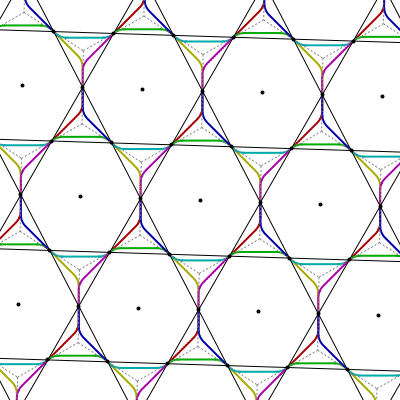Posted by: Greg Egan on September 29, 2014 5:19 AM | Permalink | Reply to this

### Re: A Packing Pessimization Problem

I think it’s actually the 3-dimensional pessimization problem where the situation should qualify as “mysterious”. It seems like a bunch of numerology comes together in a freak way to help the ball be pessimal in 3D, and it might be the only dimension where this actually happens.

Posted by: Yoav Kallus on September 26, 2014 9:16 PM | Permalink | Reply to this

### Re: A Packing Pessimization Problem

Posted by: John Baez on September 27, 2014 8:20 AM | Permalink | Reply to this

### Re: A Packing Pessimization Problem

Here’s a visualisation of what happens if you take a packing of the plane with regular hexagons, and apply the elements along a suitable path through $\mathrm{SL}_2(\mathbb{R})$.As Thomas Hales explained, the images of the vertices of the central hexagon trace out the border of the smoothed octagon in six pieces. I’ve coloured the six segments differently to make this clearer. Initially, I was a bit puzzled as to how this could work, since it’s not obvious how to divide the perimeter of an octagon into six parts. But if you divide each of the eight sides in two, along with the eight rounded corners there are twenty four parts, and each vertex of the hexagon traces out four of them.

As well as generating the shape of the smoothed octagon, this path through $\mathrm{SL}_2(\mathbb{R})$ also gives us the 1-parameter family of optimal packings, with the images of suitable points in the original hexagonal packing giving us the lattice points.

The path can be described as follows. First define:

$\phi_0(\theta)=\left( \begin{array}{cc} 1 & -\frac{\tan (\theta )}{\sqrt{3} (\tan (\theta )-1)} \\ -\tan (\theta ) & \frac{\tan ^2(\theta )+\left(2\sqrt{2}-1\right) \left(\tan (\theta )-1\right)}{\sqrt{3} (\tan (\theta )-1)} \\ \end{array} \right)$

$\theta_0 = \tan^{-1} (1-\frac{1}{\sqrt{2}})$

$\rho(\alpha) = \left( \begin{array}{cc} \cos (\alpha ) & -\sin (\alpha ) \\ \sin (\alpha ) & \cos (\alpha ) \\ \end{array} \right)$

Then the path we want is:

$\phi(\theta)=\left(\frac{\sqrt{3}}{\sqrt{2 \sqrt{2}-1}}\right) \left\{ \begin{array}{ll} \phi_0(\theta) & \quad 0 \leq \theta \leq \theta_0 \\ \rho(\pi/4) \phi_0(\theta-\theta_0) \rho(-\pi/3) & \quad \theta_0 \leq \theta \leq 2\theta_0 \\ \rho(\pi/2) \phi_0(\theta-2\theta_0) \rho(-2\pi/3) & \quad 2\theta_0 \leq \theta \leq 3\theta_0 \\ \rho(3\pi/4) \phi_0(\theta-3\theta_0) \rho(-\pi) & \quad 3\theta_0 \leq \theta \leq 4\theta_0 \end{array} \right.$

The essence of this is in $\phi_0$, which can be found by tweaking the construction I used earlier to get the shape of the smoothed octagon’s corners. The rest is just stitching and rotating four copies of that, so that the images of the six hexagon vertices give the required 24 pieces of the border.

Posted by: Greg Egan on September 27, 2014 11:52 AM | Permalink | Reply to this

### Re: A Packing Pessimization Problem

I’ve changed the image from one based on a packing of the plane solely with hexagons to one based on a packing of the plane with hexagons and triangles. The same $A_2$ lattice underlies everything, but this way there’s a single hexagon associated with every smoothed octagon.

Posted by: Greg Egan on September 27, 2014 6:30 PM | Permalink | Reply to this

### Re: A Packing Pessimization Problem

I enjoy looking at the animations in this post, and I would like to be able to generate the svg image of the path the centers take.

I think it would be fun to make a physical board. The board can have the paths routed out with a cnc machine. Smoothed octagons could be laser cut, with holes in the center for pegs. I have access to the equipment and can learn to use it, but I do not know how to generate the svg files to feed to the equipment.

For a less precise version, I could cut out smoothed octagon tiles based on the svg image on the wikipedia page, and physically trace out paths with the tiles. I will ask friends who know more if that will suffice.

For my background – I do not have mathematica, but I know python and can probably get help from friends for re-implementing code using scipy and related python tools like matplotlib. I’m not entirely confident about that, and would like to know if that seems plausible.

Posted by: Sheila on September 27, 2014 4:45 PM | Permalink | Reply to this

### Re: A Packing Pessimization Problem

Are you hoping the friction between the smoothed octagons will be low enough that as you turn one or two around, the rest will follow suit and their centers will move along the paths cut out in the board?

When my friend Matt McIrvin first saw the rotating smoothed octagons, he said he imagined a horrible screeching sound. Friction might be a problem.

Posted by: John Baez on September 27, 2014 5:23 PM | Permalink | Reply to this

### Re: A Packing Pessimization Problem

Yes, I’d like to be able to rotate everything. I think this could be a fun toy, if not that, then a nice piece of art. I could have long enough pegs to tie strings to, or things I haven’t even thought of yet.

I might be able to handle the friction problem by polishing the edges a lot and selecting an appropriate material. I have access to a laser cutter at my hackerspace that can cut or engrave different materials: paper, wood, acrylic, etc.

I haven’t through through which materials I’d use. I was thinking to cut a few trials with balsa wood or paper to see if I am going about this all wrong.

There are also other approaches to take. For example, I could try using a 3d printer and smooth out the result with acetone.

Depending on the material the friction might not be too bad?

Posted by: Sheila on September 28, 2014 1:22 AM | Permalink | Reply to this

### Re: A Packing Pessimization Problem

How would you get the octagons to rotate? (other than giving each a synchronized motor).

It may be possibler to turn each into a toothed gear (which involves even more math) and then put something like a big rubber band around a group of them to keep them in contact while the centers move around.

Posted by: RodMcGuire on September 27, 2014 5:48 PM | Permalink | Reply to this

### Re: A Packing Pessimization Problem

Alas, they’re turning in the wrong direction for toothed gears to work!

Posted by: Greg Egan on September 27, 2014 6:47 PM | Permalink | Reply to this

### Re: A Packing Pessimization Problem

For the first attempt, I imagine a handle on one octagon. For another attempt I might go with a servo.

Posted by: Sheila on September 28, 2014 1:23 AM | Permalink | Reply to this

### Re: A Packing Pessimization Problem

one might fit a size-$\varepsilon$ communicating pinion between adjacent octagons; can’t guess how stable such would be. There are also “paradoxical” (parallel worm) gears.

Posted by: Jesse C. McKeown on September 28, 2014 11:45 PM | Permalink | Reply to this

### Re: A Packing Pessimization Problem

This is what the paths of the centres look like. This image is an SVG, and if you inspect the element on the web page you’ll see that it’s basically just a list of coordinates for a lot of points on each curve. (Fancier methods are possible where shapes are approximated with Bezier curves rather than line segments.)

You can compute as many points on these curves as you want by using the matrix $\phi(\theta)$ described here, applied to points of the form $(i, \sqrt{3} j)$ for integers $i$ and $j$, followed by a counterclockwise rotation around the origin by the angle that the vector $\phi(\theta) (1,0)$ makes with the $x$ axis; this undoes the rotation of the octagons around the central one in that version of the motion. You can get the outline of one smoothed octagon (at a scale that’s compatible with these curves) by applying $\phi(\theta)$ to each of the six vertices of a regular hexagon of radius 1, with vertices $(cos (k \pi/3), sin (k \pi/3))$ for $k=0,1,2,3,4,5$.

So getting SVG files for these things is the easy part. Getting any kind of physical version of this to work sounds like the hard part to me!

Posted by: Greg Egan on September 28, 2014 1:50 AM | Permalink | Reply to this

### Re: A Packing Pessimization Problem

I wrote:

… applied to points of the form $(i, \sqrt{3} j)$ for integers $i$ and $j$

Sorry, that should be:

… applied to points of the form $(2i+j, \sqrt{3} j)$ for integers $i$ and $j$

Posted by: Greg Egan on September 30, 2014 8:29 AM | Permalink | Reply to this

### Re: A Packing Pessimization Problem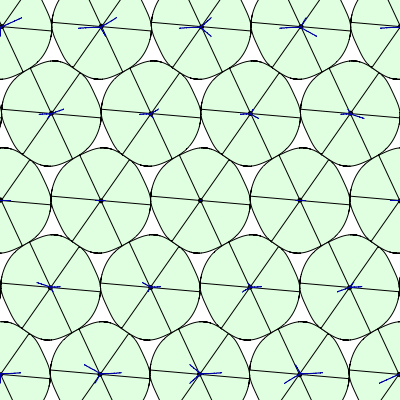I was curious as to whether there’s a (non-pessimal) shape with a 1-parameter family of equally dense lattice packings where the lattice points follow linear paths as the shape rotates.

The answer seems to be: only piecewise-linear, as in this example.

Posted by: Greg Egan on September 30, 2014 12:16 PM | Permalink | Reply to this

### Re: A Packing Pessimization Problem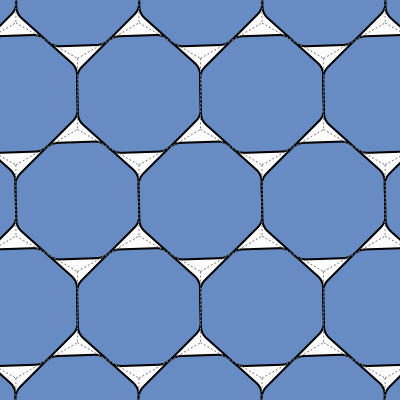This is a 1-parameter family of distorted smoothed octagons that all have the same area, the same optimal packing density, and the same best packing: an $A_2$ lattice packing, corresponding to a tiling of the plane with regular hexagons. This family of shapes is produced by acting on the ordinary smoothed octagon with the inverse of the path $\phi$ through $\mathrm{SL}_2(\mathbb{R})$ that maps the vertices of a regular hexagon to the boundary of the ordinary smoothed octagon (described here). In this image, those “generating vertices” are the midpoints of the edges of the regular hexagons that tile the plane.

Posted by: Greg Egan on September 29, 2014 9:29 AM | Permalink | Reply to this

Post a New Comment